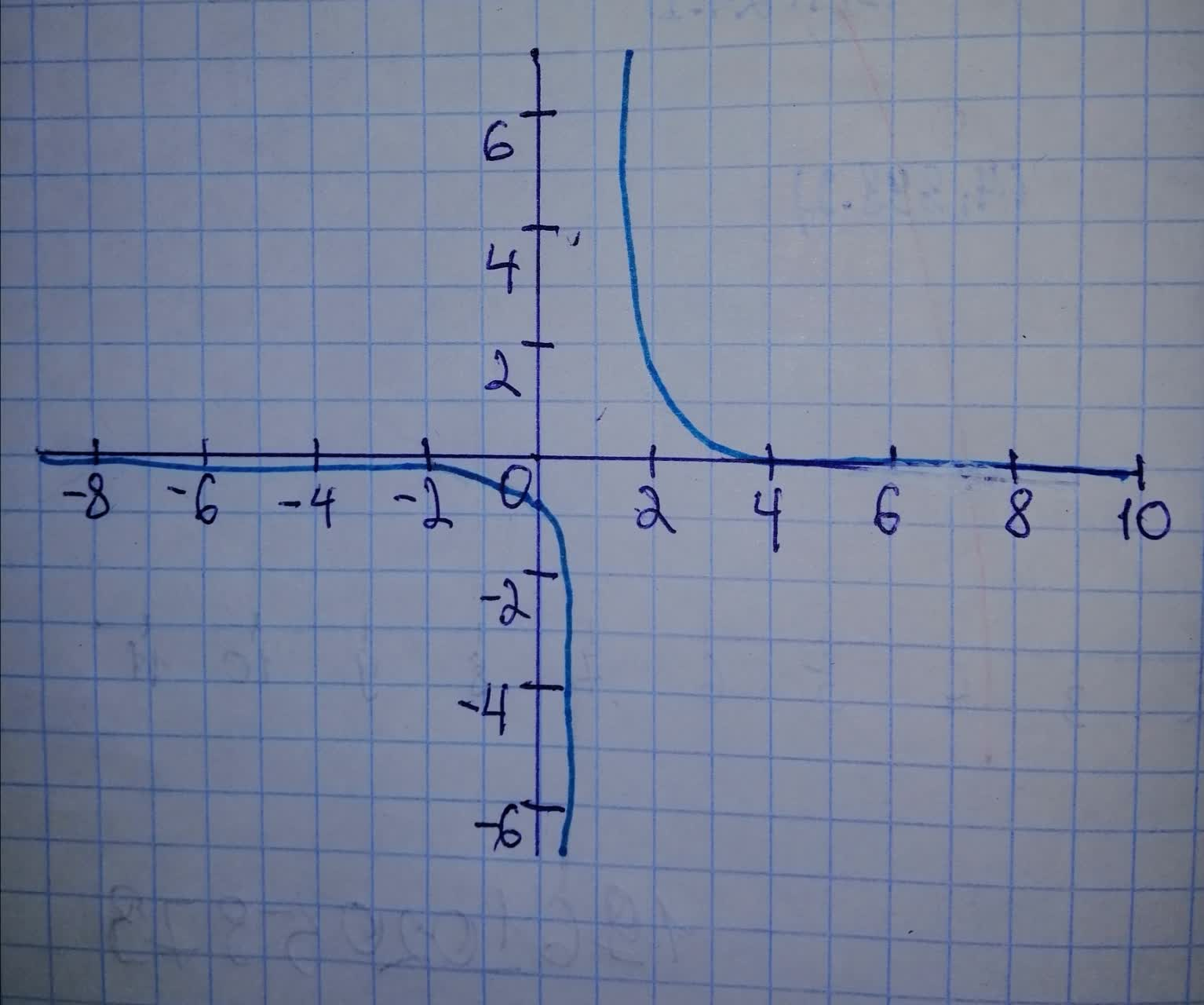Ask question

# Sketch a graph of the function. Use transformations of functions when ever possible. f(x)= frac{1}{(x - 1)^{3}}# Sketch a graph of the function. Use transformations of functions when ever possible. f(x)= frac{1}{(x - 1)^{3}}

Question
Transformations of functionsasked 2021-02-03
Sketch a graph of the function. Use transformations of functions when ever possible. $$\displaystyle{f{{\left({x}\right)}}}=\ {\frac{{{1}}}{{{\left({x}\ -\ {1}\right)}^{{{3}}}}}}$$

## Answers (1)2021-02-04
Shown is the graph of $$\displaystyle{f{{\left({x}\right)}}}=\ {\frac{{{1}}}{{{\left({x}\ -\ {z}\right)}^{{{3}}}}}}\ \text{x is on the horizontal axis and}\ {y}={f{{\left({x}\right)}}}$$ is on the vertical axis.### Relevant Questionsasked 2021-02-25
Sketch a graph of the function. Use transformations of functions when ever possible. $$\displaystyle{f{{\left({x}\right)}}}=\ {\frac{{{1}}}{{{3}}}}{\left({x}\ -\ {5}\right)},\ {2}\ \leq\ {x}\ \leq\ {8}$$asked 2021-01-02
Sketch a graph of the function. Use transformations of functions when ever possible. $$\displaystyle{f{{\left({x}\right)}}}=\ -{\frac{{{1}}}{{{x}^{{{2}}}}}}$$asked 2020-12-07
Sketch a graph of the function. Use transformations of functions when ever possible. $$\displaystyle{f{{\left({x}\right)}}}=\ -{\frac{{{1}}}{{{4}}}}{x}^{{{2}}}$$asked 2020-12-01
Sketch a graph of the function. Use transformations of functions when ever possible.
$$\displaystyle{f{{\left({x}\right)}}}={2}{x}^{{{2}}}-{1}$$asked 2020-10-20
Sketch a graph of the function. Use transformations of functions when ever possible.
$$\displaystyle{f{{\left({x}\right)}}}={\left|{x}+{1}\right|}$$asked 2021-02-05
Sketch a graph of the function. Use transformations of functions when ever possible. $$\displaystyle{f{{\left({x}\right)}}}={1}\ -\ \sqrt{{{x}\ +\ {2}}}$$asked 2021-01-16
Sketch a graph of the function. Use transformations of functions when ever possible. $$\displaystyle{f{{\left({x}\right)}}}=\sqrt{{{3}}}{\left\lbrace-{x}\right\rbrace}$$asked 2021-05-04
For each of the following functions f (x) and g(x), express g(x) in the form a: f (x + b) + c for some values a,b and c, and hence describe a sequence of horizontal and vertical transformations which map f(x) to g(x).
$$\displaystyle{f{{\left({x}\right)}}}={x}^{{2}}+{3},{g{{\left({x}\right)}}}={x}^{{2}}-{6}{x}+{8}$$asked 2021-02-06
g is related to one of the six parent functions. (a) Identify the parent function f. (b) Describe the sequence of transformations from f to g. (c) Sketch the graph of g by hand. (d) Use function notation to write g in terms of the parent function f.$$\displaystyle{g{{\left({x}\right)}}}={\frac{{{1}}}{{{3}}}}{\left({x}-{2}\right)}^{{{3}}}$$asked 2021-06-06
For each of the following functions f (x) and g(x), express g(x) in the form a: f (x + b) + c for some values a,b and c, and hence describe a sequence of horizontal and vertical transformations which map f(x) to g(x).
$$\displaystyle{f{{\left({x}\right)}}}={x}^{{2}}-{2},{g{{\left({x}\right)}}}={2}+{8}{x}-{4}{x}^{{2}}$$
...